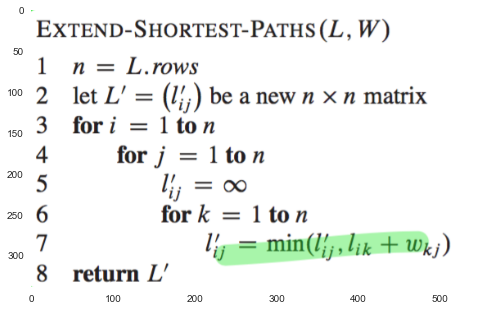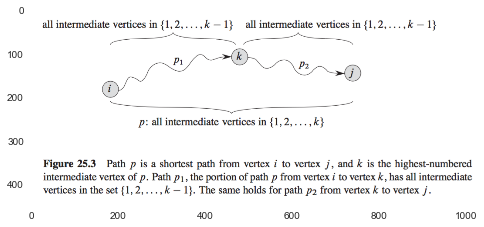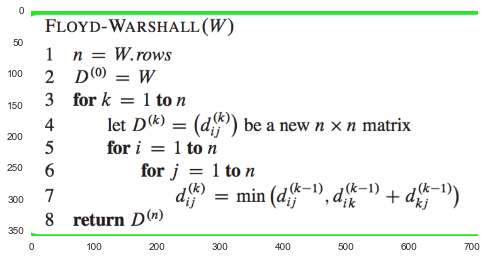In :
# %load ../../preconfig.py
%matplotlib inline
import matplotlib.pyplot as plt
import seaborn as sns
sns.set(color_codes=True)
plt.rcParams['axes.grid'] = False

#import numpy as np
#import pandas as pd

#import sklearn

#import itertools

import logging
logger = logging.getLogger()


# 25 All-Pairs Shortest Paths¶

### 25.1 Shortest paths and matrix multiplication¶

This section presents a dynamic-programming algorithm:

1. Characterize the structure of an optimal solution. all subpaths of a shortest path are shortest paths.

2. Recursively define the value of an optimal solution. let $l_{i, j}^{(m)}$ be the minimum weight of any path from vertex $i$ to vertex $j$ that contains at most $m$ edges. Thus, \begin{equation}

l_{i, j}^{(0)} = \begin{cases}
0 & \quad \text{if } i = j \\
\end{cases}


\end{equation}

we recursively define: \begin{equation}

l_{i, j}^{(m)} = \displaystyle \min_{1 \leq k \leq n} \{ l_{i, k}^{(m-1)} + w_{k, j} \}


\end{equation}

3. Compute the value of an optimal solution in a bottom-up fashion. We now compupte a series of matrices $L^{(1)}, L^{(2)}, \dotsc, L^{(n-1)}$, where $L^{(1)} = W$.

In :
plt.imshow(plt.imread('./res/ext_short_path.png'))

Out:
<matplotlib.image.AxesImage at 0x116f44ef0>If we replace $\infty$ by 0 in EXTEND-SHORTEST-PATHS, it can be converted to matrix muplication: $L^{(n-1)} = W^{n-1}$.

We can compute $L^{(n-1)}$ by divide-and-conquer methods: $O(n) \to$O(\lg n)$. Total running time:$O(n^3 n) \to O(n^3 \lg n)$In : # Exercises  ### 25.2 The Floyd-Warshall algorithm¶ use a different dynamic-programming formulation to solve.$O(V^3)$. #### The structure of a shortest path¶ intermediate vertex of a simple path$p = <v_1, v_2, \dotsc, v_n>$is any vertex of$p$other than$v_1$or$v_n$. Consider all paths from$i$to$j$whose intermediate vertices are all drawn from$\{1, 2, \dotsc, k\}$, and let$p$be a minimum-weight path from among them. • If$k$is not an intermediate vertex of path$p$, then all intermediate vertices of path$p$are in the set$\{1, 2, \dotsc, k-1\}$. • If$k$is an intermediate vertex of path$p$, then we decompose$p$into$i \overset{p_1}{\to} k \overset{p_2}{\to} j$, so all intermediate vertices of$p_1$are in the set$\{1, 2, \dotsc, k-1\}$, same with$p_2$. In : plt.imshow(plt.imread('./res/fig25_3.png'))  Out: <matplotlib.image.AxesImage at 0x11735fcc0>#### A recursive solution to the all-pairs shortest-paths problem¶ Let$d_{i j}^{(k)}$be the weight of a shortest path from vertex$i$to vertex$j$for which all intermediate vertices are in the set$\{1, 2, \dotsc, k\}$. \begin{equation} d_{i j}^{(k)} = \begin{cases} w_{i j} & \quad \text{if } k = 0 \\ \min(d_{i j}^{(k-1)}, d_{i k}^{(k-1)} + d_{k j}^{(k-1)}) & \quad \text{if } k \geq 1 \end{cases} \end{equation} #### Computing the shortest-path weights bottom up¶ In : plt.imshow(plt.imread('./res/floyd_warshall.png'))  Out: <matplotlib.image.AxesImage at 0x1187b0a90>#### Constructing a shortest path¶ #### Transitive closure of a directed graph¶ to determine whether$G$contains a path from$i$to$j$for all vetex pairs$i, j \in V$. This method subsitutes the logical OR and logical AND for the arithmetic operations$\min$and$+$in the Floyd_Warshall algorithm. In : #Exercises  ### 25.3 Johnson's algorithm for sparse graphs¶ • if all edge weight$w$in a graph$G = (V, E)$are nonnegative, find shortest paths by running Dijkstra's algorithm once from each vertext; • if$G\$ has negative-weight edges but no negative weight cycles, we use reweighting to convert it to a graph of nonegative edges.

In [ ]:
#Exercises